Next: Calculating the Equation of Up: Equations of Motion Previous: The Lagrangian

## The Orbits

The paths, or rather, the orbits of the galaxies are given in a somewhat different manner in the AVP compared with equation (3.2); namely P-89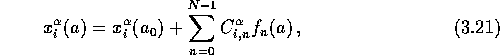where the superscriptrepresents the spatial dimensions;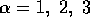(not to be confused with the parameter from Hamilton's principle). By comparing equation (3.2) with equation (3.21), one notice a few distinct differences--modifications of Hamilton's principle introduced by P-89. Firstly, the scale factor is used as a measure of time, which is justified due to the close link between time and scale factor. This is common practise in cosmology. Secondly, the first term on the right hand side in (3.2) is time dependent, while the corresponding term in (3.21) is not. The result of this alteration is that the term no longer represents the correct path in the AVP as it did in equation (3.2), but rather the present positions of the particles. Thirdly, the orbits are parameterized in the AVP picture; i.e. they are built up by the sum in the second term of equation (3.21). Theused in Hamilton's principle is in the AVP replaced by N coefficients,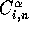, and by summing up the product of these coefficients and their respective functions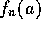, one gets an estimate of the particle orbits.

What is similar, however, are the functions used in the second term of both equation (3.2) and (3.21). Theused in the AVP, often referred to as trial functions, have some of the same characteristics as thefunction used in equation (3.2): they are both twice differentiable and continuous in the interval of the action. But, when it comes to the constraints given at the end-points, the two principles differ somewhat once again. While thein Hamilton's principle is forced to vanish at both end-points, the trial functionsonly need to vanish at the end-point representing the present time. The constraint at the other end-point, representing the initial time, is that the derivate ofwith respect to time vanishes. This is a result of the mixed boundary condition introduced by P-89, as discussed in Section 3.1. More details on these boundary conditions in the following subsection.

Now, let us take a closer look at the shape of these trial functions. The choice of functions can be arbitrary, provided that they are complete enough to make a fit of a gravitationally evolving density field between the two end-points; i.e. to mimic the deformation of the path. In this thesis, two different types of trial functions have been used; the fairly simple P-89,D-L,B-C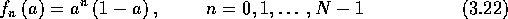and the more complex Bernoulli distribution, P-90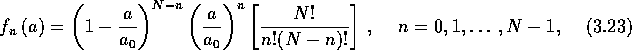where the expansion factor, a, is being normalized to unity at the present time;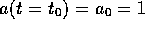. The type of trial functions has very little effect on the solution. However, as pointed out by Gia, it does have an effect on the computation of the solution, at least for systems involving a large number of particles, say, thousands of objects. With such extensive systems, considerable computing time may be saved by choosing a trial function that gives a rapid convergence of the series in equation (3.21). Peebles made use of the trial functions in equation (3.22) in his first paper dealing with the AVP P-89, but later used the Bernoulli distribution just because of its ability to speed up the computation. Other trial functions than (3.22) and (3.23) have also been used in literature, e.g. Gia,S-B,Sch used a polynomial in the linear growth function, D(t).

Equation (3.21) is only correct for an infinitely large N, which, of course, is impossible to handle numerically. Therefore, the number of terms in the series has to be finite, resulting in a discrepancy between the true orbit and the orbit obtained by the AVP. This error is referred to as ``truncation error''. For N=5, the number of correct significant digits in the positions are two, while for N=15 it has improved to six. The choice of N is a tradeoff between computing time and accuracy, and figures in literature ranges from five up to about twenty.Next: Calculating the Equation of Up: Equations of Motion Previous: The Lagrangian

Trond Hjorteland
Mon Jul 5 02:59:28 MET DST 1999